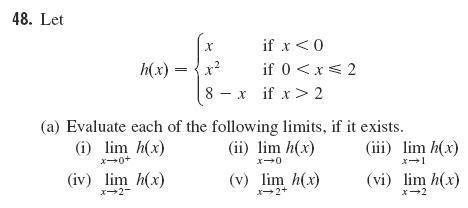# Right/left hand limit as x approaches to 0

chemic_23

## Homework Statement## Homework Equations

I trying to figure out if how will you know if which of the following h(x) will be the right/left hand limit as x approaches to 0, as x approaches to 1, as x approaches to 2? I'm confused... please help

## The Attempt at a Solution

I really don't have any idea

## Answers and Replies

To the left of 0, f(x) is identical to x. Do you know how to do $lim_{x\rightarrow 0} x$? If so, that is exactly $lim_{x\rightarrow 0^-} f(x)$.
Just to the right of 0, f(x) is identical to x2 and so the "limit from the right", $\lim_{x\rightarrow 0^+} f(x)$ is the same as $\lim_{x\rightarrow 0} x^2$.